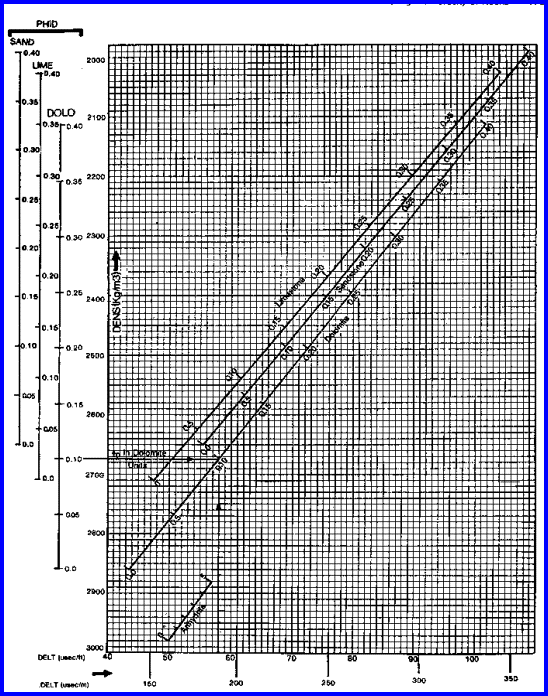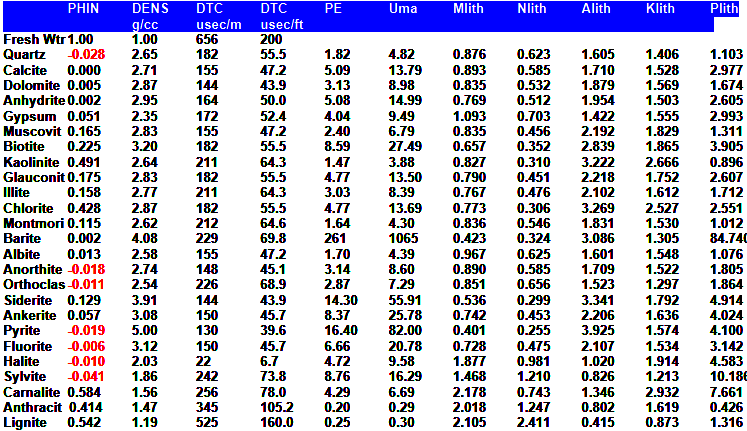Sonic DENSITY Crossplot
The sonic density crossplot model  is used to estimate porosity when the density neutron crossplot method cannot be used. The method works well when shale volume, matrix rock properties, and sonic compaction effects  are accurately known. If both density and neutron logs are available, a superior model that does not require matrix rock properties is the Shale Corrected Density Neutron Complex Lithology Crossplot Method. The Meta/Kwik spreadsheet for this model is available atSonic DENSITY CrossplOT POROSITY
The sonic density crossplot method involves the simultaneous solution of the sonic and density response equations for porosity. They are similar in form to the density neutron pair, and will not be repeated here.

The sonic density crossplot works best in shaly sands with no gas. The resolution is poor in carbonates and gas will make the result too high. The equations are shown graphically below the math.

Calculate compaction correction.
1: KCP = max (1, DTCSH / (100 + 228 * (IF DEPTHUNIT\$ = "METRIC")))

Calculate sonic shale porosity and total porosity.
2: PHISSH = (DTCSH - DTCMA) / (DTCW - DTCMA) / KCP
3: PHIS = (DTC - DTCMA) / (DTCW - DTCMA) / KCP

Calculate effective porosity:
4: PHIxsd = (PHID * PHISSH - PHIS * PHIDSH) / (PHISSH - PHIDSH)

Where:
DTCSH = sonic shale value for compaction correction (usec/ft or usec/m)
KCP = compaction factor (fractional)
DTC = sonic log reading (usec/ft or usec/m)
DTCMA = travel time in rock matrix (usec/ft or usec/m)
DTCSH = sonic log reading in shale (usec/ft or usec/m)
DTCW = travel time in water (usec/ft or usec/m)
PHID = density log reading (fractional)
PHIDSH = density shale point (fractional)
PHIS = porosity from sonic log (fractional)
PHISSH = sonic porosity in shale (fractional)
PHIxsd = porosity from density sonic crossplot (fractional)COMMENTS:
This method is pictured in below.Chart for Sonic Density Porosity Model - shale corrected data must be entered

This method is analogous to the density neutron shaly sand method. It does not work well in mixed lithology, such as limestone, dolomite and anhydrite mixtures. This method also does not use the volume of shale (Vsh) determined by the analyst, but uses the implicit shale correction determined by the sonic shale point (PHISSH) and the density shale point (PHIDSH). The sonic density crossplot should not be used in gas zones, since both logs can read too high due to gas effect.

If a matrix offset is required for the density log, use the method described in HERE.RECOMMENDED PARAMETERS: Range Default PHIDSH -0.03 to +0.20 0.00 DTCSH (English) 75 to 140 100 DTCSH (Metric) 225 to 460 328Porosity From the Sonic Density Log (Hunt-Raymer Method)
Calculate shale corrected density and sonic log readings and convert to English units.
5: PHIdc = PHID - Vsh * PHIDSH
6: DTCc = (DTC - Vsh * (DTCSH - DTCMA)) / KX2
7: DENSc = PHIdc * KD1 + (1 - PHIdc) * KD2

Where:
KD1 = 1.00
KD2 = 2.65 for Sandstone Units
KD2 = 2.71 for Limestone Units
KX2 = 3.281 for Metric Units
KX2 = 1.00 for English Units

Calculate velocity data from sonic travel time data.
8: VELOGc = 10 ^ 6 / DTCc
9: VELMA = 10 ^ 6 / DTCMA
10: VELW = 10 ^ 6 / DTCW

Calculate sonic porosity.
11: C = 1 - (VELOGc / (VELMA * ((DENSMA / DENSc) ^ 0.5))) ^ (1 / 1.9)
12: D = DTCc ^ 2 - DENSc * (DTCMA ^ 2) / DENSMA
13: E = DENSc * (DTCW ^ 2) / DENSW - DENSc * (DTCMA ^ 2) / DENSMA
14: IF C <= 0.37
15: THEN PHIxhr = C
16: OTHERWISE PHIxhr = ((0.47 - E / D) / 0.1) * E / D + ((0.37 - C) / 0.1) * C

Where:
C = intermediate term
D = intermediate term
DTC = sonic log reading in zone of interest (usec/ft or usec/m)
DTCc = sonic log reading corrected for shale (usec/ft or usec/m)
DTCMA = sonic log reading in l00% matrix rock (usec/ft or usec/m)
DTCSH = sonic log reading in l00% shale (usec/ft or usec/m)
DTCW = sonic log reading in 100% water (usec/ft or usec/m)
DENSc = density log reading corrected for shale (gm/cc)
DENSMA = matrix density (gm/cc)
E = intermediate term PHID = density log reading in zone of interest (fractional)
PHIDSH = density log reading in 100% shale (fractional)
PHIxhr = porosity from sonic log by Hunt-Raymer crossplot (fractional)
VELOGc = sonic velocity log reading corrected for shale (ft/sec or m/sec)
VELMA = sonic velocity log reading in l00% matrix rock (ft/sec or m/sec)
VELW = sonic velocity log reading in 100% water (ft/sec or m/sec)
Vsh = shale volume (fractional)COMMENTS:
The Hunt-Raymer equations for density sonic crossplot porosity are an extension of their work for the sonic log. The results are too high in gas and can be corrected by a proper choice of DENSW and DELTW. The method is not universally applicable and should be tested in each area before use.RECOMMENDED PARAMETERS:

Range       Default
PHIDSH -0.03 to +0.20 0.00
DELTSH (English) 75 to 140    100
DTCSH (Metric) 225 to 460       328NUMERICAL EXAMPLE:
1. Sonic Density Crossplot - data from Sand "D"
DTC = 300 usec/m
PHID = 0.12
DTCSH = 328 usec/m
PHIDSH = 0.03
Vsh = 0.33
DTCW = 616
DTCMA = 182
KCP = 1.00
no matrix offset
PHISSH = (328 - 182) / (616 - 182) / 1.0 = 0.33

PHIS = (300 - 182) / (616 - 182) / 1.0 = 0.27
PHIxsd = (0.12 * 0.33 - 0.27 * 0.03) / (0.33 - 0.03) = 0.105

Vsh was 0.48 using this data, but Vsh from GR is only 0.33. Therefore, porosity may be too low due to the shale correction built into this method.

2. Hunt-Raymer Sonic Density Crossplot - data from Sand "D"
Vsh = 0.33
PHIdc = 0.12 - 0.33 * 0.03 = 0.11
DTCc = (300 - 0.33 * (328 - 182)) / 3.28 = 76.8 usec/ft
DENSc = 0.11 * 1.00 + (1 - 0.11) * 2.65 = 2.47
VELOGc = 10 ^ 6 / 76.8 = 13020 ft/sec
VELMA = 10 ^ 6 / 55.5 = 18020 ft/sec
VELW = 10 ^ 6 / 188.0 = 5320 ft/sec
C = 1 - (13020 / (18020 * ((2.65 / 2.47) ^ 0.5))) ^ (1 / 1.9) = 0.173
PHIxhr = 0.173

This is considerably higher than the standard sonic density crossplot which over corrected for shale in this example.MATRIX ROCK PROPERTIESPage Views ---- Since 01 Jan 2015
Copyright 2023 by Accessible Petrophysics Ltd.
CPH Logo, "CPH", "CPH Gold Member", "CPH Platinum Member", "Crain's Rules", "Meta/Log", "Computer-Ready-Math", "Petro/Fusion Scripts" are Trademarks of the Author